## Machine Learning using Python

### Venue : RND Consultancy Services, Serampore, Chatra, Hooghly, WB. Phone : +919830546476 / +918240819346

On completion of the training, participants will be able to do data analysis using Python. Audience will be able to identify between classification and regression problem in real life data. Participants will be able to do some real life prediction like email spam filtering, stock market price prediction, loan prediction, crime data analysis etc using some machine learning tools in python.

No experience is required; however basic understanding of Python and some Basic Mathematics is highly required.

## Lesson: 1

The three different types of machine learning

Supervised, unsupervised, reinforcement learning

An introduction to the basic terminology and notations

A roadmap for building machine learning systems

Using Python for machine learning

## Lesson: 2

Introduction to Python language

Environmental setup for Python, Anaconda, Jupyter, Spider etc

Data types of Python, numbers, string

Run a python program in jupyter/spider/ipython

if, elif, loops and function in python

Module, exception of Python

## Lesson: 3

Different types of Data structure list, tuple, dictionary, set etc

What is List comprehension

Different functions of List, Set, Dictionary, Tuple

push and pop on Set

Difference between different data structures

## Lesson: 4

Introduction to Numpy and Panda

Creating Numpy array and its different operations

Slicing and indexing of Numpy Array

Introduction to Panda and Data frame

Programs using Panda Data frame

Data visualization using Matplotlib

## Lesson: 5

Dealing with missing data

Handling categorical data

Partitioning a dataset in training and test sets

Selecting meaningful features

Reduce dimensionality using PCA (Principal Component Analysis)

## Lesson: 6

What is regression

Simple Linear Regression and Multiple Linear Regression

Regression example by Decision Tree Regressor

Regression using Random Forest Regressor

## Lesson: 7

Choosing a classification algorithm

First steps with scikit-learn

Modeling class probabilities via logistic regression

Solving nonlinear problems using a kernel SVM

Decision Tree and Random Forest Classifier

K-Nearest Neighbors – a lazy learning algorithm

Text based classification using Naïve Bayes algorithm

## Lesson: 8

Unsupervised learning and clustering

Different types of unsupervised learning

K-means clustering in python

Hierarchical clustering

Difference between hierarchical and K means clustering

## Lesson: 9

Data Science overview

Data Analytics overview

Introduction to Natural language processing using Scikit learn

Uses of Tensor Flow and Open CV in data science problem

#### FIND US

Serampore, Chatra, Hooghly, West Bengal, India.
Phone: +919830546476 / +918240819346

E-mail: rndconsultancyservice@gmail.com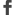Facebook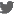Twitter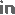LinkedInGoogle+Flicker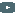YoutubePinterest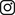Instagram